# On Development of Analytical Approach for Analysis of Energy Transfer of Traveling Wave Tube

On Development of Analytical Approach for Analysis of Energy Transfer of Traveling Wave Tube

Pankratov Evgeny Leonidovich

Nizhny Novgorod State University, 23 Gagarin avenue, Nizhny Novgorod, 603950, Russia

Nizhny Novgorod State Technical University, 24 Minin Street, Nizhny Novgorod, 603950, Russia

Corresponding Author Email:
elp2004@mail.ru
Page:
455-459
|
DOI:
https://doi.org/10.18280/mmep.060318
10 January 2019
|
Accepted:
4 June 2019
|
Published:
15 September 2019
| Citation

OPEN ACCESS

Abstract:

In this paper we introduce an analytical approach for analysis of operating regimes of traveling wave tube type O. Based on this approach we analyzed the energy transfer of the considered tube. Based on this analysis we formulate conditions to increase efficiency of this traveling wave tube.

Keywords:

traveling wave tube, analysis of operating regimes, analysis of energy transfer, increasing of efficiency, analytical approach for analysis

1. Introduction

At the present time, intensive development of sources of electromagnetic radiation (gyrotrons, ortrons, traveling wave tube, power amplifiers also develop) could be found [1-5]. It is attracted an interests increasing power of emitting of eletromagnetic radiation, increasing of electronic efficiency, decreasing of dimensions of devices, development of mathematical approaches to improve prognosis of operating of the considered devices [6-15]. In this paper we introduce an analytical approach for analysis of processes in traveling wave tube type O and determination of operation conditions of the tube (see Figure 1). Based on this approach, the energy transfer of the considered traveling wave tube and the possibility of increasing its efficiency were analyzed.

## 1.pngFigure 1. Structure of the considered traveling wave tube. B is the focusing magnetic field

2. Method of Solution

For analysis of processes taking place in traveling wave tube, the greatest interest is in the study of the motion of ultrarelativistic electrons in a traveling wave field with a constant amplitude, which we shall consider equal to

$E_{z}=-E_{0} \sin \left(k_{z} z-\omega t\right)$,       (1)

where z is the coordinate, kz is the projection of the wave number on the Oz axis, t is the time, w is the radiation frequency, and E0 is the field amplitude. At relativistic electron velocities, it is more convenient to use the relative electron energy γ, equal to the ratio of the electron energy e=mc2 to the rest energy e0=m0c2, m0 is the rest mass. From the law of conservation of energy

$y=1+e U / m_{0} c^{2}$,       (2)

where e is the electron charge, and U is the accelerating potential difference. The change in the electron energy under the action of the field in this case is described by the following equation

$\frac{d \gamma}{d z}=\frac{e}{m_{0} c^{2}} \frac{d U}{d z}=-\frac{e E_{z}}{m_{0} c^{2}}=\frac{e E_{0}}{m_{0} c^{2}} \sin \left(k_{z} z-\omega t\right)$.       (3)

We introduce a new variable: the phase of the electrons with respect to the radio-frequency field J  =kzz- w t. The equation for describing the phase is represented in the following form

$\frac{d \vartheta}{d z}=k_{z}-\omega \frac{d t}{d z}=k_{z}-\frac{\omega}{\mathcal{v}_{ \|}}$        (4)

As a result, to describe the transfer of energy to the traveling wave tube, we obtain two equations (3) and (4). We assume that the electron energy is γ = γ0(1+u), where γ0 is the energy of the particle at the entrance to the system, u is the relative changing in energy under the action of the field. We express $\mathcal{v}_{ \|}$ through γ0 and u

$\mathcal{v}_{ \|}=c \sqrt{1-\gamma^{-2}}=c \sqrt{1-\gamma_{0}^{-2}(1+u)^{-2}}$.

The relative change in energy under the action of the field is often small. In this case we can assume that

$\mathcal{v}_{ \|} \approx c \sqrt{1-\frac{1-2 u}{\gamma_{0}^{2}}}=c \sqrt{k_{z}^{2}+\frac{2 u}{\gamma_{0}^{2}}} \approx c k_{z}\left(1+\frac{u}{\gamma_{0}^{2} k_{z}^{2}}\right)$.

Substitution of this relation into equation (4) leads to the following result

$\frac{d \vartheta}{d z}=k_{z}-\frac{\omega}{c k_{z}\left(1+u \gamma_{0}^{-2} k_{z}^{-2}\right)} \approx k_{z}-\frac{\omega}{v_{1}}\left(1-\frac{u}{\gamma_{0}^{2} k_{z}^{2}}\right)=k_{z}-\frac{\omega}{\mathcal{v}_{ \|}}+\frac{\omega u}{\gamma_{0}^{2} k_{z}^{2} \mathcal{v}_{ \|}}$.

We transform the last equation so that the coefficient of u is equal to unity and introduce the dimensionless length x =z w kz3/ γ02c and the dimensionless detuning of the synchronism D=-( kz-w/ $\mathcal{v}_{ \|}$) c γ02/kz3w. Then the equation for the phase was reduced to the following form

$d \vartheta / d \xi=u-\Delta$.     (4a)

Substituting the relation γ = γ0(1+u) into relation (3), we obtain

$d u / d \xi=F \cdot \sin (\vartheta)$.      (5)

where F=kz3 eE0γ0/w m0c, u is the relative changing of energy under influence of field. The latter equations are supplemen-ted by boundary conditions. We assume that the electron beam at the input (x=0) is stationary and monoenergetic. Then the boundary conditions are written in the form

$u(0)=0 ; \vartheta(0)=\vartheta_{0}, \vartheta_{0} \in[0,2 \pi]$.         (6)

Let us now find the expression for the electronic efficiency h. The latter can be defined as the ratio of the energy increment averaged over the initial phases J0 to the original electron energy

$\eta(\xi)=\left\langle\frac{\varepsilon(\xi)-\varepsilon(0)}{\varepsilon(0)}\right\rangle$        (7a)

The last relation, taking into account the relation γ = γ0(1 +u), takes the following form

$\eta(\xi)=\frac{1}{2 \pi} \int_{0}^{2 \pi} u(\xi) d \vartheta_{0}$.        (7b)

3. Discussion

In this section we analyze the dependence of the electronic efficiency on several parameters. Figure 2 shows the dependence of the efficiency on the relative change in energy under the action of the field. The figure shows monotonous increasing of this dependence. In Figure 3 shows the dependence of the efficiency on the dimensionless length with explicit maximum.

## 2.png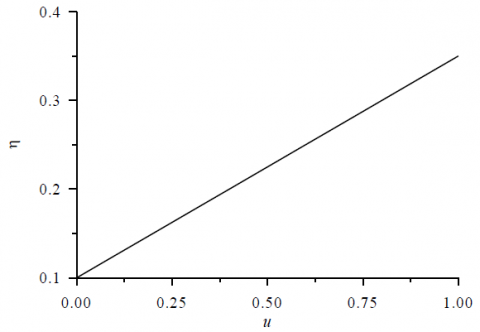Figure 2. Dependence of the efficiency on the relative change in energy under the action of the field

## 3.png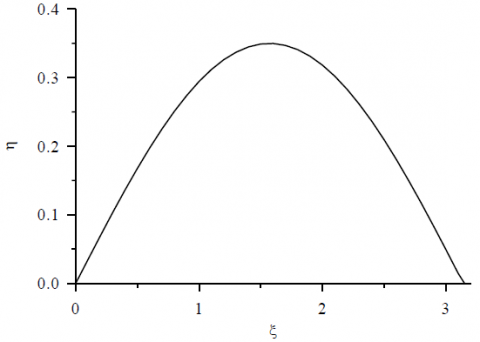Figure 3. Dependence of the efficiency of a dimensionless length

## 3.pngFigure 4. Dependence of relative electron energy γ on accelerating potential difference U

The Figure 4 and 5 show dependence of relative electron energy on accelerating potential difference U and rest energy e0. These figures show linear increasing and hyperbolic decreasing of the considered relative electron energy. Both dependences are enough natural.

## 5.pngFigure 5. Dependence of relative electron energy γ on rest energy e0

Velocity $\mathcal{v}_{ \|}$ increases with increasing of the above relative electron energy γ (see Figure 6). Analogous dependence could be found as a function of the energy on relative changing in energy under the action of field u (see Figure 7).

## 6.png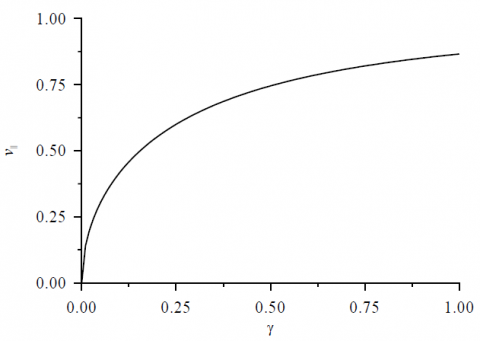Figure 6. Dependence of velocity $\mathcal{v}_{ \|}$ on relative electron energy γ

## 7.png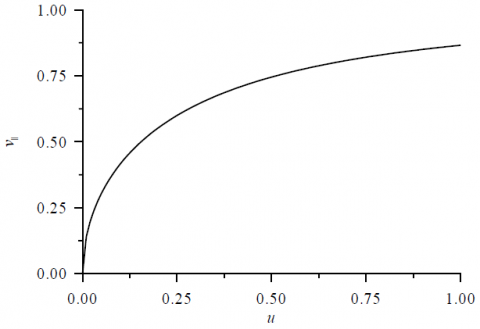Figure 7. Dependence of velocity $\mathcal{v}_{ \|}$ on relative changing in energy under the action of field u

Dimensionless detuning of the synchronism D decreasing at absolute value with increasing of wave number kz (see Figure 8), leads to the constant value with increasing of frequency w (see Figure 9), decreasing at absolute value with decreasing of velocity $\mathcal{v}_{ \|}$ (see Figure 10).

## 8.pngFigure 8. Dependence of dimensionless detuning of the synchronism D on wave number kz

## 9.pngFigure 9. Dependence of dimensionless detuning of the synchronism D on frequency w

## 10.pngFigure 10. Dependence of dimensionless detuning of the synchronism D on velocity $\mathcal{v}_{ \|}$

Dimensionless length x  increases with increasing of frequency w became also unlimited with linear increasing (see Figure 11) and with cubic nonlinearity as function of wave number kz (see Figure 12).

## 11.pngFigure 11. Dependence of dimensionless length x on frequency w

## 12.png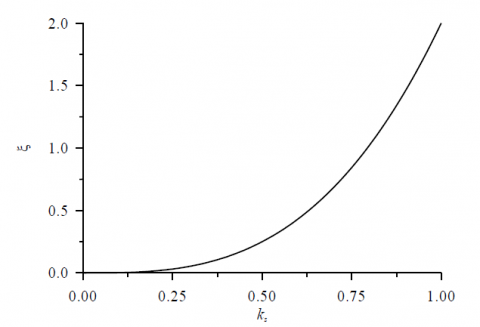Figure 12. Dependence of dimensionless length x on wave number kz

## 13.png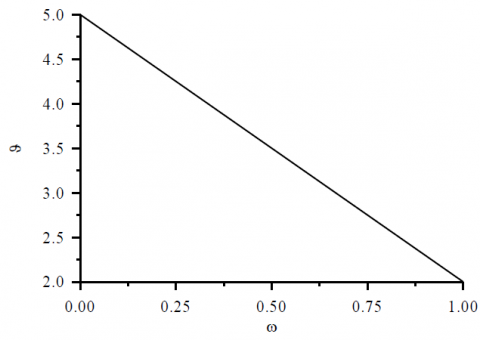Figure 13. Dependence of phase of electrons J on frequency w

## 14.pngFigure 14. Dependence of phase of electrons J on wave number kz

Phase of the considered electrons with respect to the radio- frequency field J has linear increasing with increasing of wave number kz (see Figure 12) and linear increasing with increasing of frequency w (see Figure 13).

4. Conclusion

In this paper we introduce an analytical approach for analysis of operating regimes of traveling wave tube type O. Based on this approach we analyzed the energy transfer of the considered tube. Based on this analysis we formulate conditions to increase efficiency of this traveling wave tube. For example, efficiency of traveling wave tube could be increased with increasing of energy of electromagnetic field. Efficiency of this tube also depends on its length. But this dependence is nonmonotonous and has a single maximal value.

References

 Perel’stein, E.A., Bobyleva, L.V., Elzhov, A.V., Kazacha, V.I. (1999). Theoretical investigation of the bunching of an electron beam in relativistic power amplifiers. Technical Physics, 44(2): 222-225. http://dx.doi.org/10.1134/ 1.1259288

 Ginzburg, N.S., Zavol’sky, N.A., Zapevalov, V.E., Moiseev, M.A., Novozhylov, Y.V. (2000). Nonstationary processes in a diffraction-output orotron. Technical Physics, 45(4): 480-485. http://dx.doi.org/10.1134/1.1259659

 Kostienko, A.I., Korolev, A.F., Pereshein, V.M. (1991). Quasi-optical gyrotron with a three-mirror telescopic resonator. Bulletin of Moscow State University Series, 3: Physics, Astronomy, 32(2): 23-28.

 Azov, G., Ganeev, E., Khritkin, S. (2016). Centimeter spiral traveling wave tube: design methods and development results. Electronics Science, Technology, Business 1: 155-159.

 Andreevskaya, T.M. (2014). About the possibility of simultaneous amplification of several powerful broadband TWT television channels. T-Comm Issue, 10: 6-9.

 Klimov, A.V., Manuilov, V.N. (2019). Numerical modeling of analyzer of parameters of electron beams of powerful gyrotrons. Advances in Applied Physics, 7(1): 63-69.

 Manuilov, V.N., Morozkin, M.V., Glyavin, M.Y., Luksha, O.I. (2018). Gyrotron collector systems: Types and capabilities. Infrared Physics & Technology, 91(1): 46-54.

 Zavolsky, N.A., Ilyakov, E.V., Kalynov, Y.K., Kulagin, I.S., Manuilov, V.N., Shevchenko, A.S. (2018). High-power relativistic milimeter-wave gyrotron operating at the second cyclotron harmonic. Radiophysics and Quantum Electronics, 60(1): 40-47. http://dx.doi.org/10.1007/ s11141-018-9868-5

 Manuilov, V.N., Samsonov, S.V., Mishakin, S.V., Klimov, A.V., Leshcheva, K.A. (2018). Cusp guns for helical-waveguide gyro-twts of a high-gain high-power w-band amplifier cascade. Journal of Infrared, Millimeter and Terahertz Waves, 39(5): 447-455. http://dx.doi.org/10.1007/s10762-018-0473-7

 Kalynov, Y.K., Manuilov, V.N., Zaslavsky, V.Y. (2018). Influence of perturbations in the axial symmetry on formation of helical electron beams in a system with the reversed magnetic field. Journal of Infrared, Millimeter, and Terahertz Waves, 39(8): 738-748. http://dx.doi.org/10.1007/s10762-018-0508-0

 Ginzburg, N.S., Abubakirov, E.B., Vilkov, M.N., Zotova, I.V., Sergeev, A.S. (2018). Generation of a Periodic Sequence of high-power ultrashort pulses in a chain of coupled backward-wave and traveling-wave tubes operating in the regimes of amplification and nonlinear Kompfner suppression. Technical Physics, 88(8): 1205-1211. http://dx.doi.org/10.1134/S1063784218080078

 Ginzburg, N.S., Kocharovskaya, E.R., Vilkov, M.N., Sergeev, A.S. (2018). Using multichannel laser complexes for incoherent pumping of x-ray compton free-electron lasers. Technical Physics Letters, 44(7): 605-608. http://dx.doi.org/10.1134/S1063785018070210

 Vilkov, M.N., Ginzburg, N.S., Zotova, I.V., Sergeev, A.S. (2018). Scenarios for inclusion of ultracurot pulses generator based on two related screw gyro-traveling wave tube working in amplifier and nonlinear absorption modes. Bullutein of the Russian Academy of Sciences. A series of Physics, 82(1): 61-66.

 Zaslavsky, V.Y., Ginzburg, N.S., Zotova, I.V., Sergeev, A.S. (2018). Stabilization of the azimutal structure of the pul-ses of cherenkovskaya radiation when using two dimensional grinded extensive cylindrical waveges. Electronics and Microelectronics Microwaves, 1: 201-204.

 Abubakirov, E.B., Ginzburg, N.S., Zotova, I.V., Leont'ev, A.N., Malkin, A.M., Rozental', R.M., Sergeev, A.S. (2018). Overlay of the spectra of the chaotic generation of neighbored modes in a high-current relativistic gyrotron. Electronics and Microelectronics of Microwave, 1: 699-701.